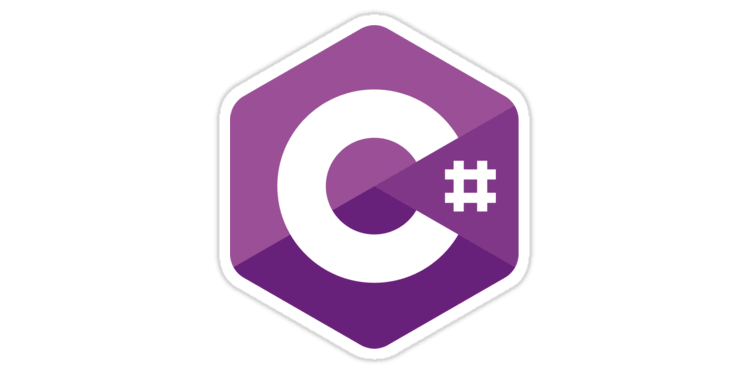# How to check if a number is a multiple of another in ASP.NET C#A minimalistic extension method written in C Sharp that can be used to determine if an integer is a multiple (or a dividend) of another integerToday I needed to find a quick way to programmatically determine if a given integer number (Int32) was a multiple of another integer number: as a matter of fact I needed it to build a grid-based HTML structure, but I guess that there are a ton of scenarios when such feature might be handy.

For that very reason, I've built a very simple helper class containing a couple extension methods that can be used to check if a given int (we'll call it multiple) is a multiple of another int (dividend): to determine that I've used the the modulus operator (%), which returns the remainder after dividing two numbers: if the remainder is 0, it means that multiple is evenly divisible by dividend.

Here's the extension method class:

As we can see, there are two methods that are based upon the same logic:

• IsMultipleOf(), which returns TRUE if the former int is a multiple of the latter, FALSE otherwise.
• IsDividendOf(), which is basically the reciprocal of the previous one, which returns TRUE if the former int is a dividend of the latter, FALSE otherwise.

If you don't know how to use the above Extension Methods in a real-case scenario, read this article.

In case you don't like Extension Methods, here's the same code written as a static helper class:

## Conclusions

That's all: I hope that this minimalistic set of extension methods will help other developers looking for a quick and effective way to check if a number is a number (or a dividend) or another.